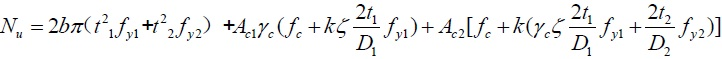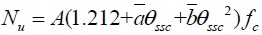Table. 1: Ultimate bearing capacity formulas of the composite CFST short columns.

Theoretical method No. Ultimate bearing capacity formulas Section
Limit equilibrium theory  1a
Modified formulas based on limit equilibrium theory [4, 10, 11] 2a
34Twin-shear unified strength theory [9, 12] 5a, b
6a,b
The unified theory of CFST  7a, b
Notes:
1. η represents the discount coefficient that considering the desynchronized limit load of the inner and outer steel tube, which generally takes the value of 0.9; α1, α2 respectively represents the confinement coefficient of the outer and inner concrete. The computational methods of the above three parameters are seen in reference .
2. Ac1, Ac2, As1, and As2 respectively represents the area of the outer concrete, the inner concrete, the outer steel tube, the inner steel tube and the area of the total column cross-section; fc, fy1, fy2 respectively represents the axial compressive strength of the concrete and the yield strength of the outer and inner steel tube; t1, t2 and D1, D2 respectively represents the thickness and the diameter of the outer and inner steel tube. When the outer steel tube is square, t1 and D1 can be equally transformed to the thickness and the diameter of the circular tube according to the principle of equivalent area for the outer concrete and steel tube.
3. θ1, θ2 respectively represents the confinement index of the outer and inner concrete and the expressions are: θ1= fy1As1/fcAc1 and
θ2= fy2As2/fcAc2.
4. βf represents the strength discount coefficient of the steel tube under limit state; γc represents the strength discount coefficient of the concrete when the square steel tube is equally transformed to the circular steel tube. As for the circular steel tube, γc equals to 1.0; krepresents the side-pressure coefficient; In view of the influence of the ratio of the edge length and the thickness, ζ represents the discount coefficient of the uneven confinement that the square steel tube set on the concrete. As for the circular steel tube, ζ equals to 1.0; p1 represents the confining stress the outer steel tube set on both the inner concrete and the outer concrete, p2 represents the confining stress the inner steel tube set on the inner concrete; b in formula (6) represents a weighting parameter, which reflects the influences of the middle shear stress and the corresponding normal stress on the yield or destruction of the material. The computational details of the above parameters are seen in reference .
5. θssc in formula (7) represents the composite equivalent confinement coefficient;are the impact parameters of the steel and concrete. The definition and the detailed calculation are seen in reference .
6. The subscripts a and b represent the cross section of the composite short columns in Fig. (1).